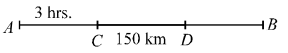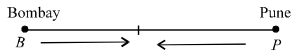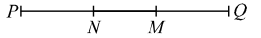## Speed, Time and Distance

#### Speed, Time and Distance

1. A train met with an accident 3 hours after starting, which detains it for one hour, after which it proceeds at 75% of its original speed. It arrives at the destination 4 hours late. Had the accident taken place 150 km further along the railway line,
 the train would have arrived only 3 1 hours late. 2
Find the length of the trip and the original speed of the train.
1. 1100 km ; 100 kmph
2. 1200 km ; 100 kmph
3. 1200 km ; 90 kmph
4. 1600 km ; 90 kmph

1. Let A be the starting point, B the terminus. C and D are points where accidents take place.∴ 0.75 = 3 4

By travelling a 3/4 of its original speed, the train would take 4/3 of its usual time i.e., 1/3 more of the usual time.
∴  1/3 of the usual time taken to travel the distance CB.
= 4 – 1 = 3 hrs.     ...(i)
and 1/3 of the usual time taken to travel the distance
 DB = 3 1 − 1 = 2 1 hrs.   ...(ii) 2 2

Subtracting equation (ii) from (i)
we can write,
1/3 of the usual time taken to travel the distance
 CD = 3 − 2 1 = 1 hr. 2 2

∴  Usual time taken to travel
 CD (150 km) = 1 2 1 3

 = 3 hr. 2

Usual speed of the train
 = 3 = 9 hrs. 1 3

Total time = 3 + 9 = 12 hrs.
∴  Length of the trip = 12 × 100 = 1200 km.

##### Correct Option: B

Let A be the starting point, B the terminus. C and D are points where accidents take place.∴ 0.75 = 3 4

By travelling a 3/4 of its original speed, the train would take 4/3 of its usual time i.e., 1/3 more of the usual time.
∴  1/3 of the usual time taken to travel the distance CB.
= 4 – 1 = 3 hrs.     ...(i)
and 1/3 of the usual time taken to travel the distance
 DB = 3 1 − 1 = 2 1 hrs.   ...(ii) 2 2

Subtracting equation (ii) from (i)
we can write,
1/3 of the usual time taken to travel the distance
 CD = 3 − 2 1 = 1 hr. 2 2

∴  Usual time taken to travel
 CD (150 km) = 1 2 1 3

 = 3 hr. 2

Usual speed of the train
 = 3 = 9 hrs. 1 3

Total time = 3 + 9 = 12 hrs.
∴  Length of the trip = 12 × 100 = 1200 km.

1. A train meets with an accident after travelling 30 kms, after which it moves
 with 4 th of its original speed and arrives 5
at the destination 45 minutes late. Had the accident happened 18 kms further on, it would have been 9 minutes before. Find the distance of journey and original speed of the train.
1. 120 km ; 25 kmph
2. 125 km ; 25 kmph
3. 130 km ; 30 kmph
4. 120 km ; 30 kmph

1. Let the original speed be x and distance be y
Case I.
Time taken by train to travel

 30 km = 30 x

 Time taken by train after accident = y − 30 4/5x

 Total time taken = 30 + y − 30 x 4/5x

Case II :
Time taken by train to travel
 48 km = 48 x

 Time taken by train after accident = y − 48 4/5x

 Total time taken = 48 + y − 48 x 4/5x30 + y − 30−48 + y − 48x 4/5x x 4/5x

 = 9 [∵ Difference between time is 9 minutes] 60y − 30 − y − 48+30 − 484/5x 4/5x x x

 = 9 60

 y − y − 30 + 48 + (− 18) = 9 4/5x x 60

 5(18) − 18 = 9 4x 4x 60

 ⇒ 90 − 72 = 9 4x 60

 x = 18 × 60 = 30 4 × 9

Hence, original speed = 30 kmph
Also,
 30 + y − 30 = y + 45 x 4/5x x 60

[Original time + 45 minute = New time]
⇒  3x – y = –30
⇒  3(30) – y = –30
⇒  i.e. Distance = 120 km

##### Correct Option: D

Let the original speed be x and distance be y
Case I.
Time taken by train to travel

 30 km = 30 x

 Time taken by train after accident = y − 30 4/5x

 Total time taken = 30 + y − 30 x 4/5x

Case II :
Time taken by train to travel
 48 km = 48 x

 Time taken by train after accident = y − 48 4/5x

 Total time taken = 48 + y − 48 x 4/5x30 + y − 30−48 + y − 48x 4/5x x 4/5x

 = 9 [∵ Difference between time is 9 minutes] 60y − 30 − y − 48+30 − 484/5x 4/5x x x

 = 9 60

 y − y − 30 + 48 + (− 18) = 9 4/5x x 60

 5(18) − 18 = 9 4x 4x 60

 ⇒ 90 − 72 = 9 4x 60

 x = 18 × 60 = 30 4 × 9

Hence, original speed = 30 kmph
Also,
 30 + y − 30 = y + 45 x 4/5x x 60

[Original time + 45 minute = New time]
⇒  3x – y = –30
⇒  3(30) – y = –30
⇒  i.e. Distance = 120 km

1. Ram starts his journey from Bombay to Pune and simultaneously Mohan starts from Pune to Bombay. After crossing each other they finish their remaining
 journey in 6 1 and 4 hours respectively. 4
What is Mohan’s speed if Ram’s speed is 20 km per hr. ?
1. 28 kmph
2. 24 kmph
3. 25 kmph
4. 30 kmph

1.Suppose that Ram and Mohan meet at A. Let Ram’s speed be x km per hr. and Mohan’s speed be y km per hr.

 Then AP = 25 x km x

and AB = 4y km.
Now, time taken by Ram in going from
 B to A = 4y x

and the time taken by Mohan in going from
 P to A = 25x . 4y

Obviously time taken is equal
 ∴ 4y = 25x x 4y

⇒  16y2 = 25x2
 ⇒ y2 = 25 x2 16

 ⇒ y = 5 x 4

 ⇒  y = 5 x 4

Here, x = 20 km per hr.
∴  y = Mohan’s speed
 = 5 × 20 = 25 km per hr. 4

##### Correct Option: CSuppose that Ram and Mohan meet at A. Let Ram’s speed be x km per hr. and Mohan’s speed be y km per hr.

 Then AP = 25 x km x

and AB = 4y km.
Now, time taken by Ram in going from
 B to A = 4y x

and the time taken by Mohan in going from
 P to A = 25x . 4y

Obviously time taken is equal
 ∴ 4y = 25x x 4y

⇒  16y2 = 25x2
 ⇒ y2 = 25 x2 16

 ⇒ y = 5 x 4

 ⇒  y = 5 x 4

Here, x = 20 km per hr.
∴  y = Mohan’s speed
 = 5 × 20 = 25 km per hr. 4

1. A car driving in the morning fog passes a man walking at 4 km per hr. in the same direction. The man can see the car for 3 minutes and visibility is upto a distance of 130 metres. Find the speed of the car.
1. 7.5 kmph
2. 6.6 kmph
3. 6 kmph
4. 7 kmph

1. Distance covered by man in 3 minutes

 =4 × 1000m × 3 minutes 60 minutes

=200 metres
Total distance covered by the car in 3 min.
= (200 + 130) m = 330 metres
 ∴   Speed of the car = 330 m per min. 3

= 110 m per minutes
 = 110 1000 1 60

 = 33 km per hr. 5

or 6.6 kmph

##### Correct Option: B

Distance covered by man in 3 minutes

 =4 × 1000m × 3 minutes 60 minutes

=200 metres
Total distance covered by the car in 3 min.
= (200 + 130) m = 330 metres
 ∴   Speed of the car = 330 m per min. 3

= 110 m per minutes
 = 110 1000 1 60

 = 33 km per hr. 5

or 6.6 kmph

1. A and B start simultaneously at 5 km per hr. and 4 km per hr. from P and Q, 180 kms apart, towards Q and P respectively. They cross each other at M and after reaching Q and P turn back immediately and meet again at N. Find the distance MN.
1. 45 km
2. 40 km
3. 35 km
4. 42 km

1.When A and B cross each other at M for the first time, they have together covered the whole distance PQ = 180 km.
When they meet again at N, they have together covered total distance equal to 3 times of PQ = 3 × 180 = 540 km.

 PM = 5 × 180 = 100 km 5 + 4

[Distance covered by each will be in the ratio of their speeds)
 QP + PN = 4 × 540 = 240 km 5 + 4

or PN = 240 – QP = 240 – 180 = 60 km.
Then, MN = PM – PN
= 100 – 60 = 40 km.

##### Correct Option: BWhen A and B cross each other at M for the first time, they have together covered the whole distance PQ = 180 km.
When they meet again at N, they have together covered total distance equal to 3 times of PQ = 3 × 180 = 540 km.

 PM = 5 × 180 = 100 km 5 + 4

[Distance covered by each will be in the ratio of their speeds)
 QP + PN = 4 × 540 = 240 km 5 + 4

or PN = 240 – QP = 240 – 180 = 60 km.
Then, MN = PM – PN
= 100 – 60 = 40 km.